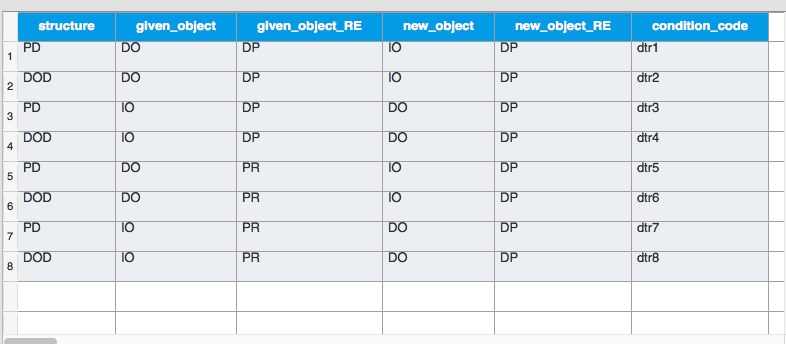#### Howdy, Stranger!

It looks like you're new here. If you want to get involved, click one of these buttons!

Supported by

# different examples per condition

Hi All,

I have created a full factorial loop design with 8 conditions total. I need to have each condition 4 times. However, I cannot simply randomise and repeat as each example (8 conditions x 4 examples) will have to be different, i.e contain a different context sentence and thus also a different target (I am measuring reaction times on whether the target sentence is considered 'good or 'bad'). How can I implement 4 different examples per each condition?
Thank you.

• Hi,

It sounds like you can treat 'example' as a factor in your design, for example through a variable `example_nr`. See this tutorial for a similar situation:

Does that work for you?

Cheers,
Sebastiaan

There's much bigger issues in the world, I know. But I first have to take care of the world I know.
cogsci.nl/smathot

• Hi Sebastiaan,

My current loop looks like this:Would this mean that I would have to first multiply each condition by the how many examples I want to have per each condition (say 6) and add the 'exampleN' variable, and specify in the table the text for each example? In my case, this would include the text for the context and the text for the target sentence.
Is there a way to multiply the conditions automatically? Or do I just copy/paste the table N times?

Marta

• Would this mean that I would have to first multiply each condition by the how many examples I want to have per each condition (say 6) and add the 'exampleN' variable, and specify in the table the text for each example?

Yes, I think this is the right thing to do. Basically you need a unique line in your loop table for each example you have.

Eduard